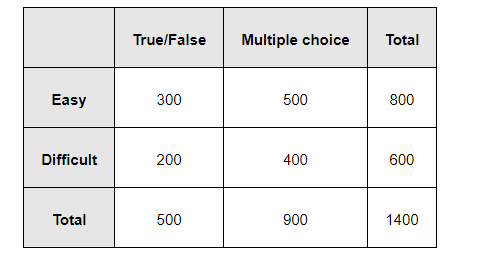# An instructor has a question bank consisting of 300 easy True/False questions,`
Question:

An instructor has a question bank consisting of 300 easy True/False questions, 200 difficult True/False questions, 500 easy multiple choice questions and 400 difficult multiple choice questions. If a question is selected at random from the question bank, what is the probability that it will be an easy question given that it is a multiple choice question?

Solution:

The given data can be tabulated asLet us denote E = easy questions, M = multiple choice questions, D = difficult questions, and T = True/False questions

Total number of questions = 1400

Total number of multiple choice questions = 900

Therefore, probability of selecting an easy multiple choice question is

$P(E \cap M)=\frac{500}{1400}=\frac{5}{14}$

Probability of selecting a multiple choice question, P (M), is

$\frac{900}{1400}=\frac{9}{14}$

P (E|M) represents the probability that a randomly selected question will be an easy question, given that it is a multiple choice question.

$\therefore \mathrm{P}(\mathrm{E} \mid \mathrm{M})=\frac{\mathrm{P}(\mathrm{E} \cap \mathrm{M})}{\mathrm{P}(\mathrm{M})}=\frac{\frac{5}{14}}{\frac{9}{14}}=\frac{5}{9}$

Therefore, the required probability is $\frac{5}{9}$.